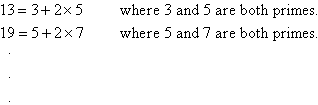Year 7 Interactive Maths - Second Edition

## Problem Solving Unit

### Problem 11.1  Levy's Conjecture

The mathematician Levy noticed in 1964 that:From this observation, Levy thought that every odd number could be expressed as a sum of one prime number and double of another prime number.

Your task is to see if this statement (called Levy's conjecture) is true for odd numbers up to 101.